Publicité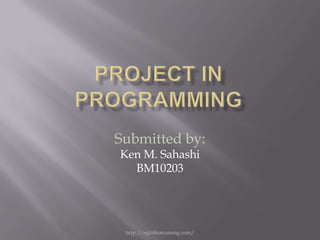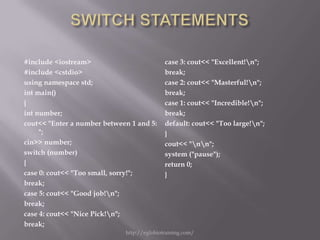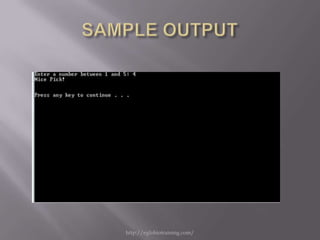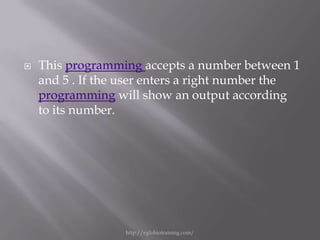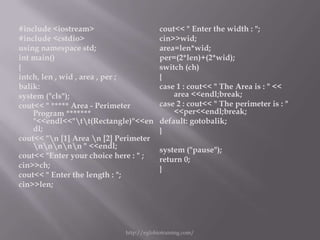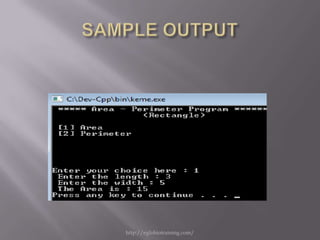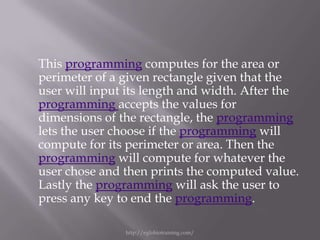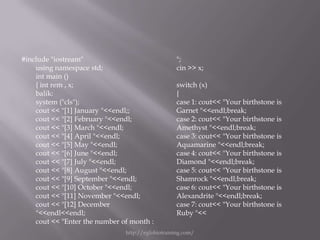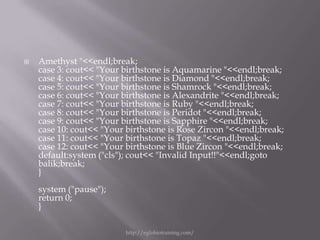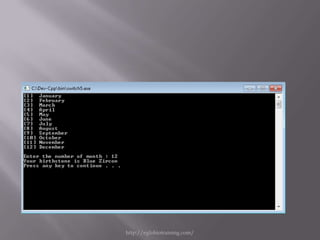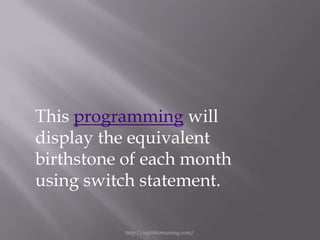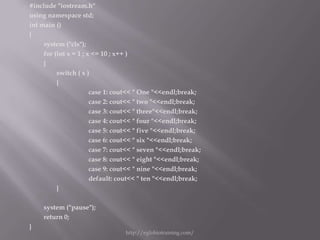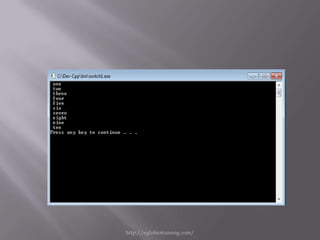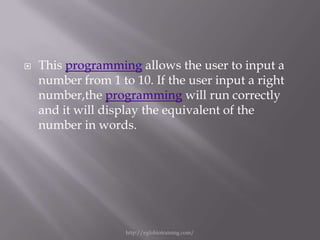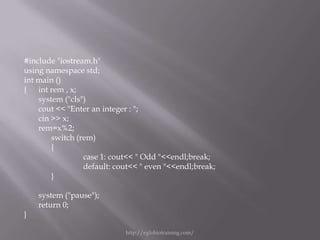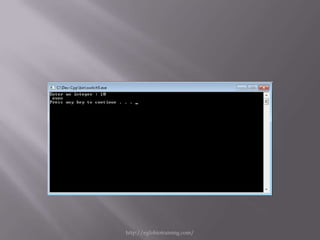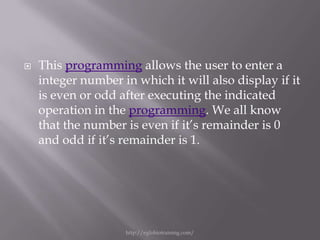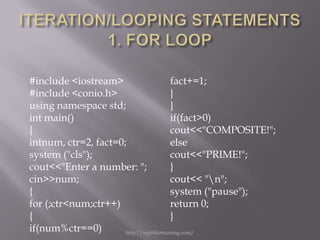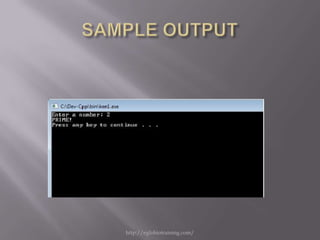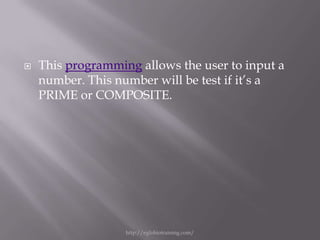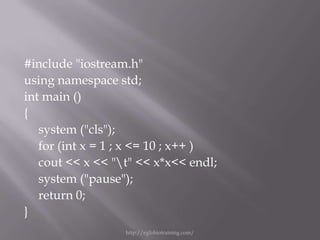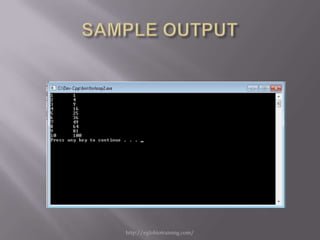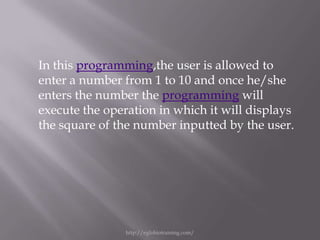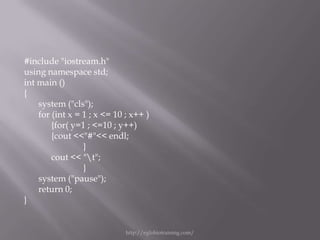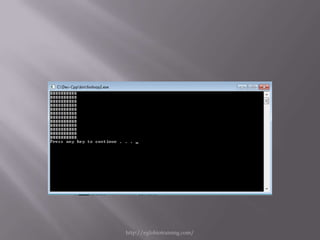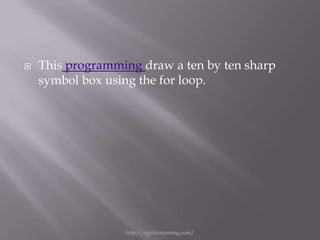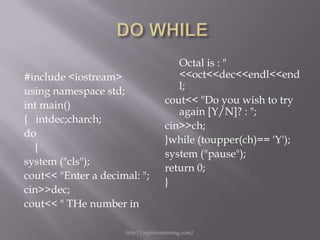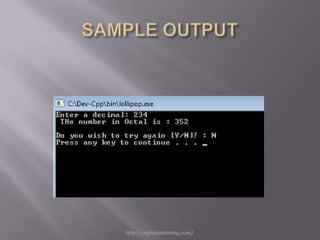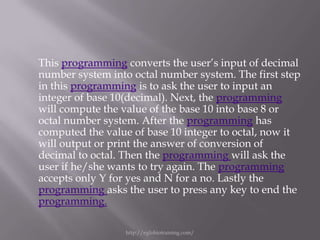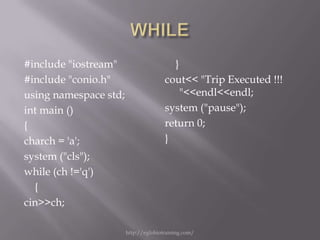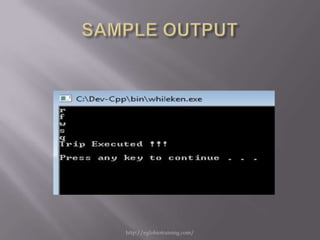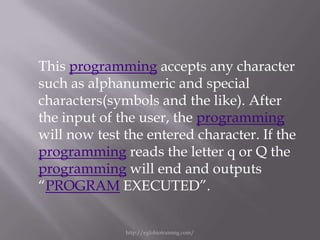1 sur 33
Publicité

### Project in programming

1. Submitted by: Ken M. Sahashi BM10203 http://eglobiotraining.com/
2. #include <iostream> case 3: cout<< "Excellent!n"; #include <cstdio> break; using namespace std; case 2: cout<< "Masterful!n"; int main() break; { case 1: cout<< "Incredible!n"; int number; break; cout<< "Enter a number between 1 and 5: default: cout<< "Too large!n"; "; } cin>> number; cout<< "nn"; switch (number) system ("pause"); { return 0; case 0: cout<< "Too small, sorry!"; } break; case 5: cout<< "Good job!n"; break; case 4: cout<< "Nice Pick!n"; break; http://eglobiotraining.com/
3. http://eglobiotraining.com/
4. This programming accepts a number between 1 and 5 . If the user enters a right number the programming will show an output according to its number. http://eglobiotraining.com/
5. #include <iostream> cout<< " Enter the width : "; #include <cstdio> cin>>wid; using namespace std; area=len*wid; int main() per=(2*len)+(2*wid); { switch (ch) intch, len , wid , area , per ; { balik: case 1 : cout<< " The Area is : " << system ("cls"); area <<endl;break; cout<< " ***** Area - Perimeter case 2 : cout<< " The perimeter is : " Program ******* <<per<<endl;break; "<<endl<<"tt(Rectangle)"<<en default: gotobalik; dl; } cout<< "n  Area n  Perimeter nnnnn " <<endl; system ("pause"); cout<< "Enter your choice here : " ; return 0; cin>>ch; } cout<< " Enter the length : "; cin>>len; http://eglobiotraining.com/
6. http://eglobiotraining.com/
7. This programming computes for the area or perimeter of a given rectangle given that the user will input its length and width. After the programming accepts the values for dimensions of the rectangle, the programming lets the user choose if the programming will compute for its perimeter or area. Then the programming will compute for whatever the user chose and then prints the computed value. Lastly the programming will ask the user to press any key to end the programming. http://eglobiotraining.com/
8. #include "iostream" "; using namespace std; cin >> x; int main () { int rem , x; switch (x) balik: { system ("cls"); case 1: cout<< "Your birthstone is cout << " January "<<endl;; Garnet "<<endl;break; cout << " February "<<endl; case 2: cout<< "Your birthstone is cout << " March "<<endl; Amethyst "<<endl;break; cout << " April "<<endl; case 3: cout<< "Your birthstone is cout << " May "<<endl; Aquamarine "<<endl;break; cout << " June "<<endl; case 4: cout<< "Your birthstone is cout << " July "<<endl; Diamond "<<endl;break; cout << " August "<<endl; case 5: cout<< "Your birthstone is cout << " September "<<endl; Shamrock "<<endl;break; cout << " October "<<endl; case 6: cout<< "Your birthstone is cout << " November "<<endl; Alexandrite "<<endl;break; cout << " December case 7: cout<< "Your birthstone is "<<endl<<endl; Ruby "<< cout << "Enter the number of month : http://eglobiotraining.com/
9. Amethyst "<<endl;break; case 3: cout<< "Your birthstone is Aquamarine "<<endl;break; case 4: cout<< "Your birthstone is Diamond "<<endl;break; case 5: cout<< "Your birthstone is Shamrock "<<endl;break; case 6: cout<< "Your birthstone is Alexandrite "<<endl;break; case 7: cout<< "Your birthstone is Ruby "<<endl;break; case 8: cout<< "Your birthstone is Peridot "<<endl;break; case 9: cout<< "Your birthstone is Sapphire "<<endl;break; case 10: cout<< "Your birthstone is Rose Zircon "<<endl;break; case 11: cout<< "Your birthstone is Topaz "<<endl;break; case 12: cout<< "Your birthstone is Blue Zircon "<<endl;break; default:system ("cls"); cout<< "Invalid Input!!"<<endl;goto balik;break; } system ("pause"); return 0; } http://eglobiotraining.com/
10. http://eglobiotraining.com/
11. This programming will display the equivalent birthstone of each month using switch statement. http://eglobiotraining.com/
12. #include "iostream.h" using namespace std; int main () { system ("cls"); for (int x = 1 ; x <= 10 ; x++ ) { switch ( x ) { case 1: cout<< " One "<<endl;break; case 2: cout<< " two "<<endl;break; case 3: cout<< " three"<<endl;break; case 4: cout<< " four "<<endl;break; case 5: cout<< " five "<<endl;break; case 6: cout<< " six "<<endl;break; case 7: cout<< " seven "<<endl;break; case 8: cout<< " eight "<<endl;break; case 9: cout<< " nine "<<endl;break; default: cout<< " ten "<<endl;break; } system ("pause"); return 0; } http://eglobiotraining.com/
13. http://eglobiotraining.com/
14. This programming allows the user to input a number from 1 to 10. If the user input a right number,the programming will run correctly and it will display the equivalent of the number in words. http://eglobiotraining.com/
15. #include "iostream.h" using namespace std; int main () { int rem , x; system ("cls") cout << "Enter an integer : "; cin >> x; rem=x%2; switch (rem) { case 1: cout<< " Odd "<<endl;break; default: cout<< " even "<<endl;break; } system ("pause"); return 0; } http://eglobiotraining.com/
16. http://eglobiotraining.com/
17. This programming allows the user to enter a integer number in which it will also display if it is even or odd after executing the indicated operation in the programming. We all know that the number is even if it’s remainder is 0 and odd if it’s remainder is 1. http://eglobiotraining.com/
18. #include <iostream> fact+=1; #include <conio.h> } using namespace std; } int main() if(fact>0) { cout<<"COMPOSITE!"; intnum, ctr=2, fact=0; else system ("cls"); cout<<"PRIME!"; cout<<"Enter a number: "; } cin>>num; cout<< "n"; { system ("pause"); for (;ctr<num;ctr++) return 0; { } if(num%ctr==0) http://eglobiotraining.com/
19. http://eglobiotraining.com/
20. This programming allows the user to input a number. This number will be test if it’s a PRIME or COMPOSITE. http://eglobiotraining.com/
21. #include "iostream.h" using namespace std; int main () { system ("cls"); for (int x = 1 ; x <= 10 ; x++ ) cout << x << "t" << x*x<< endl; system ("pause"); return 0; } http://eglobiotraining.com/
22. http://eglobiotraining.com/
23. In this programming,the user is allowed to enter a number from 1 to 10 and once he/she enters the number the programming will execute the operation in which it will displays the square of the number inputted by the user. http://eglobiotraining.com/
24. #include "iostream.h" using namespace std; int main () { system ("cls"); for (int x = 1 ; x <= 10 ; x++ ) {for( y=1 ; <=10 ; y++) {cout <<"#"<< endl; } cout << "t"; } system ("pause"); return 0; } http://eglobiotraining.com/
25. http://eglobiotraining.com/
26. This programming draw a ten by ten sharp symbol box using the for loop. http://eglobiotraining.com/
27. Octal is : " #include <iostream> <<oct<<dec<<endl<<end using namespace std; l; int main() cout<< "Do you wish to try again [Y/N]? : "; { intdec;charch; cin>>ch; do }while (toupper(ch)== 'Y'); { system ("pause"); system ("cls"); return 0; cout<< "Enter a decimal: "; } cin>>dec; cout<< " THe number in http://eglobiotraining.com/
28. http://eglobiotraining.com/
29. This programming converts the user’s input of decimal number system into octal number system. The first step in this programming is to ask the user to input an integer of base 10(decimal). Next, the programming will compute the value of the base 10 into base 8 or octal number system. After the programming has computed the value of base 10 integer to octal, now it will output or print the answer of conversion of decimal to octal. Then the programming will ask the user if he/she wants to try again. The programming accepts only Y for yes and N for a no. Lastly the programming asks the user to press any key to end the programming. http://eglobiotraining.com/
30. #include "iostream" } #include "conio.h" cout<< "Trip Executed !!! using namespace std; "<<endl<<endl; int main () system ("pause"); { return 0; charch = 'a'; } system ("cls"); while (ch !='q') { cin>>ch; http://eglobiotraining.com/
31. http://eglobiotraining.com/
32. This programming accepts any character such as alphanumeric and special characters(symbols and the like). After the input of the user, the programming will now test the entered character. If the programming reads the letter q or Q the programming will end and outputs “PROGRAM EXECUTED”. http://eglobiotraining.com/
33. This powerpoint is saved at http://www.slideshare.net/ This project is submitted to: Prof. Erwin Globio http://eglobiotraining.com/ http://eglobiotraining.com/
Publicité